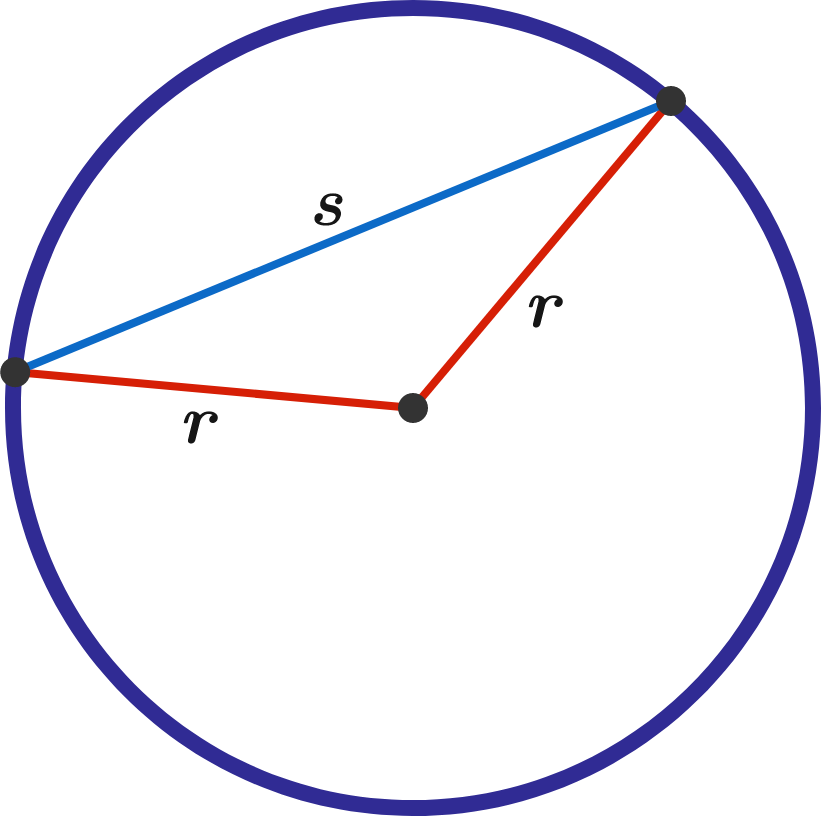# Probability that the length of chord AB is greater than the radius of a circle.

Probability Level 1

Two points are placed randomly along the circumference of a circle. What is the probability that the chord drawn between them is longer than the radius of the circle?How often is Length of Chord > Radius?

×